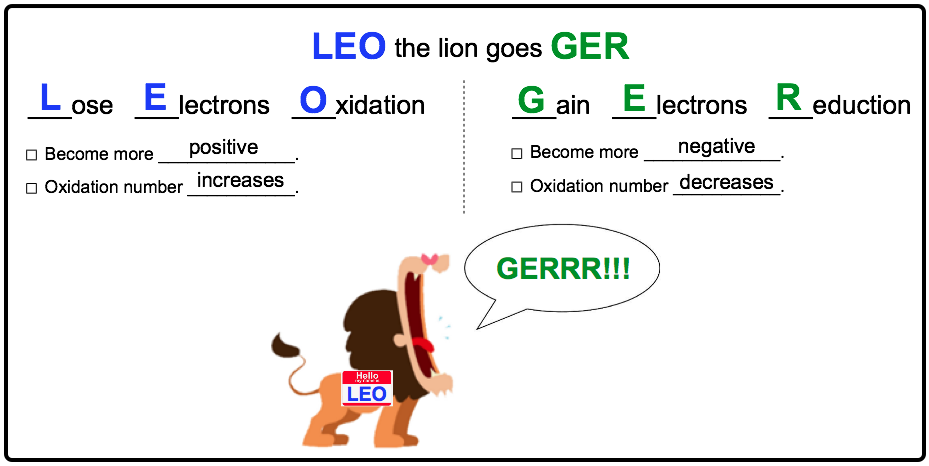Clutch Prep is now a part of Pearson
Ch.4 - Chemical Quantities & Aqueous ReactionsWorksheetSee all chapters

# Redox Reactions

See all sections
Sections
Solutions
Molarity
Osmolarity
Dilutions
Solubility Rules
Electrolytes
Molecular Equations
Gas Evolution Equations
Solution Stoichiometry
Complete Ionic Equations
Calculate Oxidation Numbers
Redox Reactions
Balancing Redox Reactions: Acidic Solutions
Balancing Redox Reactions: Basic Solutions
Activity Series

Redox Reactions (oxidation-reduction reactions) involve transference of an electron(s) between reactants.

###### Oxidation-Reduction Reactions

Concept #1: Redox Reactions

To remember the difference between oxidation and reduction just learn: LEO the lion goes GERConcept #2: Redox Reactions

Oxidizing Agent is reduced and in turn oxidizes another element/compound.

Reducing Agent is oxidized and in turn reduces another element/compound.

Example #1: Which reactant is undergoing oxidation and which reactant is undergoing reduction?

Practice: Which element is being reduced in the following reaction?

Cr2O72- + 3 HNO2 + 5 H→ 2 Cr3+ +  3NO3- + 4 H2O

Practice: Identify the oxidizing agent and reducing agent from the following redox reaction.

Ba (s) + Cl2 (g) → BaCl2 (aq)

Practice: Which element is oxidized and which is reduced in the following reaction?

Hg (aq) + HgCl2 (aq) → Hg2Cl2

Practice: Which of the following represents an oxidation-reduction reaction?

I. PCl3 (aq) + Cl2 (g) → PCl5 (aq)

II. 2 AgNO3 (aq) + Cu (s) → Cu(NO3)2 (aq) + 2 Ag (s)

III. CO2 (g) + 2 LiOH (aq) → Li2CO3 (aq) + H2O (l)

IV. FeCl2 (aq) + 2 NaOH (aq) → Fe(OH)2 (aq) + 2 NaCl (aq)